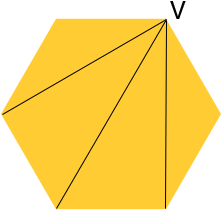Quandaries and Queries are all of diagonals in a hexagon has same length Hi Brittany, I assume that you mean a regular hexagon. I drew a diagram of a regular hexagon, chose one vertex and called it V, and then drew the three diagonals that pass through V.Two of the diagonals are of the same length but one is larger than the other two. Penny Go to Math Central